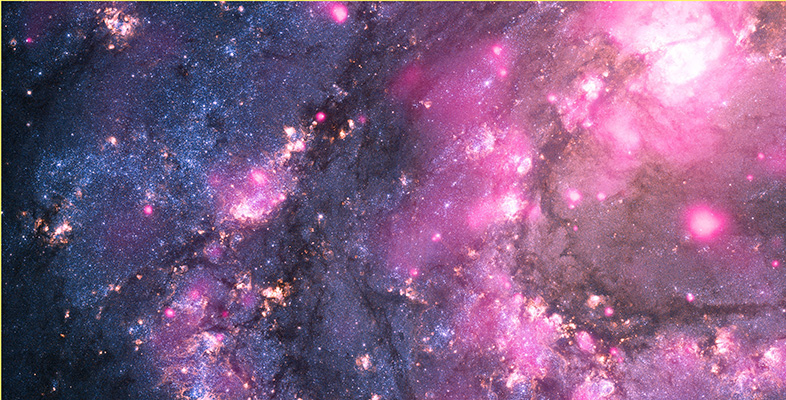The restless Universe

Start this free course now. Just create an account and sign in. Enrol and complete the course for a free statement of participation or digital badge if available.

3 Appendix: Some highlights of physics

 c. 624 BC Birth of Thales of Miletus: traditionally 'the first physicist'. 384 BC Birth of Aristotle: author of Physics. 1543 Nicolaus Copernicus' De Revolutionibus Orbium Celestium. 1600 William Gilbert's De Magnete describing the behaviour of magnets. 1609 Johannes Kepler's first and second laws published in Astronomia Nova. 1632 Galileo's Dialogue Concerning the Two Chief Systems of the World published. 1638 Galileo's work on motion described in his Discorsi. 1687 Newton's laws of motion and gravitation published in his Principia. 1704 Newton's work on light and spectra described in his Opticks. 1729 Stephen Gray discovers electrical conduction. 1736 Leonhard Euler introduces differential equations into mechanics. 1755 Euler lays the foundations of fluid mechanics. 1784 Pierre Laplace introduces concept of electric potential. 1785 Charles Coulomb announces his law of electrostatics. 1799 Pierre Laplace's Méchanique Céleste (Volume 1). 1801 Thomas Young demonstrates the wave nature of light. 1803 John Dalton proposes his atomic theory of matter. 1820 Hans Oersted demonstrates electromagnetism. 1821 Michael Faraday demonstrates the principle of the electric motor. 1825 Sadi Carnot lays the foundations of thermodynamics. 1843 James Joule determines the mechanical equivalent of heat. 1847 Hermann von Helmholtz formulates conservation of energy. 1848 Lord Kelvin proposes the absolute temperature scale. 1849 Armand Fizeau makes first accurate measurement of the speed of light. 1850 Rudolf Clausius introduces entropy. 1859 James Clerk Maxwell develops the kinetic theory of gases. 1865 Maxwell's Dynamical Theory of the Electromagnetic Field. 1871 Dmitry Mendeleev's periodic table of the elements. 1877 Ludwig Boltzmann introduces statistical interpretation of entropy. 1882 Albert Michelson measures the speed of light. 1887 Michelson-Morley experiment fails to detect the ether. 1887 Heinrich Hertz discovers photoelectric effect. 1888 Heinrich Hertz demonstrates the existence of radio waves. 1895 Wilhelm Röntgen discovers X-rays. 1896 Henri Becquerel discovers radioactivity. 1897 J. J. Thomson discovers the electron. 1900 Max Planck introduces the quantum. 1905 Einstein publishes papers on special relativity, Brownian motion and the photoelectric effect. 1911 Ernest Rutherford announces discovery of the atomic nucleus. 1911 Victor Hess discovers cosmic rays. 1913 Niels Bohr's quantum theory of the atom. 1916 Einstein's general theory of relativity. 1924 Bose-Einstein statistics introduced. 1925 Heisenberg introduces quantum mechanics (matrix form). 1925 Wolfgang Pauli announces the exclusion principle. 1926 Schrödinger introduces wave mechanics. 1926 Bom's probability interpretation of quantum mechanics. 1926 Fermi-Dirac statistics introduced. 1926 Heisenberg formulates the uncertainty principle. 1928 The Dirac equation describes relativistic electrons and leads to an understanding of spin and the prediction of antiparticles. 1929 Edwin Hubble discovers the expansion of the Universe. 1932 James Chadwick discovers the neutron. 1932 Carl Anderson discovers the positron. 1934 Fermi introduces the weak interaction. 1935 Hideki Yukawa lays the foundation of the strong interaction. 1939 Otto Hahn and Lise Meitner discover nuclear fission. 1948 John Bardeen, William Brattain and William Shockley produce the transistor. 1948 Feynman introduces his diagrams for quantum electrodynamics. 1948 George Gamow proposes the basis of Big Bang theory. 1964 Murray Gell-Mann introduces quarks. 1965 Arno Penzias and Robert Wilson discover cosmic microwave background radiation. 1967 Jocelyn Bell Burnell discovers first pulsar (a neutron star). 1968 Steven Weinberg, Abdus Salam and Sheldon Glashow develop unified theory of electroweak interaction. 1972 Fritsch, Gell-Mann and Bardeen develop quantum chromodynamics. 1977 Klaus von Klitzing discovers the quantum Hall effect. 1980 Alan Guth proposes an inflationary early Universe. 1981 Green and Schwarz introduce superstring theory. 1982 Alain Aspect conducts experiment demonstrating non-local aspects of quantum physics. 1986 Bednorz and Mueller discover high-temperature superconductivity. 1991 CERN confirms the existence of three generations of fundamental particles. 1995 Witten and Townsend develop M-Theory. 1995 Cornell, Wieman and Anderson discover Bose-Einstein condensate of atomic gas.